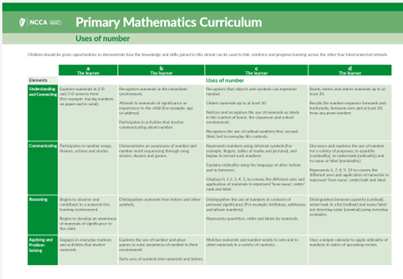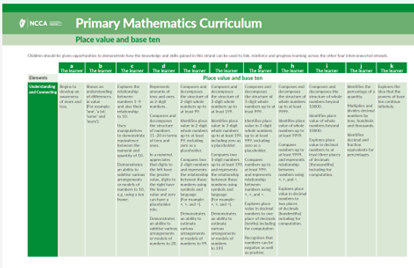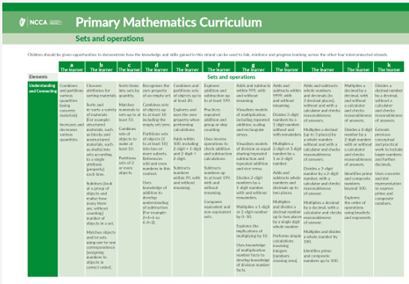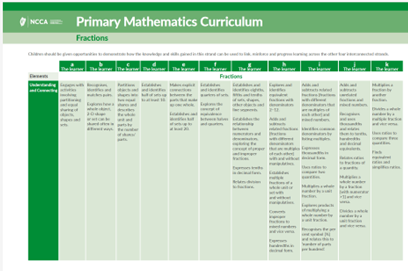NCCA uses cookies so that you have the best possible browsing experience on our website. If you agree that we can store and use cookies click 'Accept & Close'.Manage Cookies

# Number

Find below the Learning Outcomes, mathematical concepts and progression continua associated with the strand units of "Uses of Number", "Numeration and counting", "Place value and base ten", "Sets and Operations" and "Fractions". This page will be updated with further support materials and video examples of children's learning in the coming weeks and months.

### Stage 4

#### 5th & 6th Class Through appropriately playful and engaging learning experiences, children should be able to

develop an awareness that numbers have a variety of uses.

## Mathematical Concepts

 Numbers can be used in different ways. Numbers are used for labelling and identification purposes (nominality), e.g. phone number, number of a bus. Numbers denote quantity or the amount within a set (cardinality). Numbers describe the position of something in a list such as 1st, 2nd, 3rd (ordinality). Numbers are used to measure.

## Progression Continuum

Click on the image to access the progression continuum for the strand unit of 'Uses of number'### Stage 4

#### 5th & 6th Class Through appropriately playful and engaging learning experiences, children should be able to

develop an awareness that the purpose of counting is to quantify.

use a range of counting strategies for a range of purposes.

demonstrate proficiency in using and applying different counting strategies.

## Mathematical Concepts

 Quantities can be subitised and compared without needing to count or assign a numerical value. There are five principles of counting; one-one, stable order, cardinal, order irrelevance and abstraction. The last number in the count indicates the quantity in a set. There are a range of counting strategies, including grouping objects and arranging objects in various visual configurations. Estimation and counting strategies can be applied to determine quantities / calculations. The reasonableness of estimations can be tested by counting. There are a range of strategies for counting forwards and backwards.

## Progression Continuum

Click on the image to access the progression continuum for the strand unit of 'Numeration and counting'### Stage 4

#### 5th & 6th Class Through appropriately playful and engaging learning experiences, children should be able to

develop a sense of ten as the foundation for place value and counting.

understand that digits have different values depending on their place or position in a number.

use estimation to quickly determine number values and number calculations.

explore equivalent numerical expressions of numbers using the base ten system.

investigate how decimals and percentages (and fractions) can be compared, ordered and expressed in related terms.

## Mathematical Concepts

 Numbers can be distinguished according to their quantitative value. The base of our number system is ten. The base-ten number system consists of 10 digits and is based on groups of ten. In a 2-digit number, the digit to the left denotes the greater value. The value of a digit in a number depends on its place. The position of a digit denotes a value ten times that of the digit to its right. When ten place value units (e.g. ones, tens) are grouped, a new place value unit (e.g. ten, hundred) is formed. The relationship between one quantity and another quantity can be an equality or inequality relation. 0 can be used as a placeholder, allowing us to record a number accurately. Numbers can be rounded or approximated to provide estimations of value. The value of each digit in an integer or decimal number is a multiple of the value of its place. The value of an integer or decimal number is represented by the value of the sum of each of its constituent digits. The principle of base ten holds for integers and decimals. Notwithstanding the conventional notation, numbers can be represented in different, equivalent ways using concrete materials (e.g. 46 = 4 tens and 6 units or 3 tens and 16 units). A decimal point is a convention that separates the integer part of the number (left) from the fraction part of the number (right). The base ten place value system extends indefinitely in two directions multiplying (to the left) or dividing (to the right) by multiples of ten. Fractions, decimals and percentages are three ways of expressing part-whole relationships. A rational number is any number that can be written as a fraction, where both the numerator and the denominator are integers, and the denominator is not equal to zero. Multiples of 10 are a useful tool for converting between fractions, decimals and percentages. A percentage is a way of expressing a fraction of one hundred or another way of writing hundredth. Per ‘cent’ means out of a hundred and uses the % notation.

## Progression Continuum

Click on the image to access the progression continuum for the strand unit of 'Place value and base ten'### Stage 4

#### 5th & 6th Class Through appropriately playful and engaging learning experiences, children should be able to

recognise and understand what happens when quantities (sets) are partitioned and combined.

select, make use of and represent a range of addition and subtraction strategies.

understand and apply flexibly the four operations; and the relationships between operations.

build upon, select and make use of a range of operation strategies.

## Mathematical Concepts

 Quantities (or sets) can be partitioned and combined. Adding a natural number to a natural number makes the number (quantity) bigger. Subtracting a natural number from a natural number makes the number (quantity) smaller. This can be represented as a move on the number line or 100 square. A whole number does not change when adding or subtracting zero from that number. Addition and subtraction have an inverse relationship. Commutative, associative, additive identity and distributive are significant properties of addition. Numbers and symbols are used to construct and express number sentences. These can help to solve problems or are used to express contexts mathematically. When combining or partitioning numbers, we sometimes need to exchange tens to units, or hundreds to tens where necessary. A number fact is a mental picture of the relationship between a number and the parts that combine to make it. Representations of subtraction can include reduction, complement and difference. Commutative, associative,  identity and distributive properties apply to the operation of multiplication. One definition of multiplication is having a certain number of groups of the same size.  An early representation of multiplication is repeated addition. The principles used when performing operations on whole numbers are very similar for decimal numbers, with consideration needed on how to handle the decimal point. Division can be described as the splitting of a number into equal parts or groups, or the repeated subtraction of a number. Multiplication and division have an inverse relationship. Use of a calculator can reduce computative focus allowing for increased focus on strategies. Estimation and rounding are useful to test the reasonableness of answers to more complex operations. For fractional and decimal computation, new and amended algorithms are needed as some meanings of whole number operations may be difficult to apply. A prime number has exactly two factors – itself and one, a composite number has three or more factors.  The number one is neither prime nor composite. Factors are numbers that multiply together to give a product. Multiples are the result of multiplying a whole number by a whole number (or an integer by an integer).

## Progression Continuum

Click on the image to access the progression continuum for the strand unit of 'Sets and operations'### Stage 4

#### 5th & 6th Class Through appropriately playful and engaging learning experiences, children should be able to

develop an awareness of part-whole relationships using a variety of models (area, length and set).

recognise and name fractions according to their part-whole relationships.

explore the concept of equivalence in terms of simple fractions.

compare and express in equivalent terms; and order fractions.

calculate the fraction of quantities and express in multiple ways.

explore (model, compare and convert) the relationships between fractions, decimals and percentages.

investigate proportionality and ratios of quantities (sets).

## Mathematical Concepts

 Sets, objects and spaces can be partitioned in different ways. Fractions are a representation of part-whole relationships. Fractions are named according to their number of equal parts or shares. Each equal share of a set has the same value. Numbers may be expressed as numerous equivalent fractions. The greater the number of portions of a whole, the smaller the size of each portion. A numerator denotes the number of parts, the denominator denotes the total number of parts in a whole. A fraction may be considered as a representation of division. Fraction families are helpful to show how fractions are related and / equivalence, and when adding and subtracting fractions. Fractions can express value greater than one. Improper fractions have numerators that are higher than the denominators. Fractions can be more easily added / subtracted when they have a common denominator. Fractions can be represented in decimal and percentage form. Ratios can be used to compare two or more whole numbers and have corresponding representations as fractions. Multiplying or dividing a fraction by a fractional equivalent of one does not alter its value. This can be useful for exploring equivalence and / or computation involving fractions.

## Progression Continuum

Click on the image to access the progression continuum for the strand unit of 'Fractions'